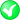#Boost C++ Libraries

...one of the most highly regarded and expertly designed C++ library projects in the world.

#### clear

Clears a linestring, ring or polygon (exterior+interiors) or multi*.

###### Description

Generic function to clear a geometry. All points will be removed from the collection or collections making up the geometry. In most cases this is equivalent to the .clear() method of a std::vector<...>. In the case of a polygon, this clear functionality is automatically called for the exterior ring, and for the interior ring collection. In the case of a point, boxes and segments, nothing will happen.

###### Synopsis

```template<typename Geometry>
void clear(Geometry & geometry)```

###### Parameters

Type

Concept

Name

Description

Geometry &

Any type fulfilling a Geometry Concept

geometry

A model of the specified concept which will be cleared

Either

`#include <boost/geometry.hpp>`

Or

`#include <boost/geometry/algorithms/clear.hpp>`

###### Conformance

The function clear is not defined by OGC.

The function clear conforms to the clear() method of the C++ std-library.

###### Supported geometries

Geometry

Status

PointSegmentBoxLinestringRingPolygonMultiPointMultiLinestringMultiPolygonVariant###### Behavior

Case

Behavior

Point

Nothing happens, geometry is unchanged

Segment

Nothing happens, geometry is unchanged

Box

Nothing happens, geometry is unchanged

Linestring

Linestring is cleared

Ring

Ring is cleared

Polygon

The exterior ring is cleared and all interior rings are removed

Multi Point

Multi Point is cleared

Multi Linestring

Multi Linestring is cleared

Multi Polygon

Multi Polygon is cleared

Constant

###### Example

Shows how to clear a ring or polygon

```#include <iostream>

#include <boost/geometry.hpp>
#include <boost/geometry/geometries/polygon.hpp>
#include <boost/geometry/geometries/ring.hpp>

BOOST_GEOMETRY_REGISTER_BOOST_TUPLE_CS(cs::cartesian)

#include <boost/assign.hpp>

int main()
{
using boost::assign::tuple_list_of;

typedef boost::tuple<float, float> point;
typedef boost::geometry::model::polygon<point> polygon;
typedef boost::geometry::model::ring<point> ring;

polygon poly;

// Fill the polygon (using its own methods + Boost.Assign)
poly.outer() = tuple_list_of(0, 0)(0, 9)(10, 10)(0, 0);
poly.inners().push_back(tuple_list_of(1, 2)(4, 6)(2, 8)(1, 2));

std::cout << boost::geometry::dsv(poly) << std::endl;
boost::geometry::clear(poly);
std::cout << boost::geometry::dsv(poly) << std::endl;

// Create a ring using Boost.Assign
ring r = tuple_list_of(0, 0)(0, 9)(8, 8)(0, 0);

std::cout << boost::geometry::dsv(r) << std::endl;
boost::geometry::clear(r);
std::cout << boost::geometry::dsv(r) << std::endl;

return 0;
}
```

Output:

```(((0, 0), (0, 10), (11, 11), (0, 0)), ((0, 0), (0, 10), (11, 11), (0, 0)))
(())
((0, 0), (0, 9), (8, 8), (0, 0))
()
```